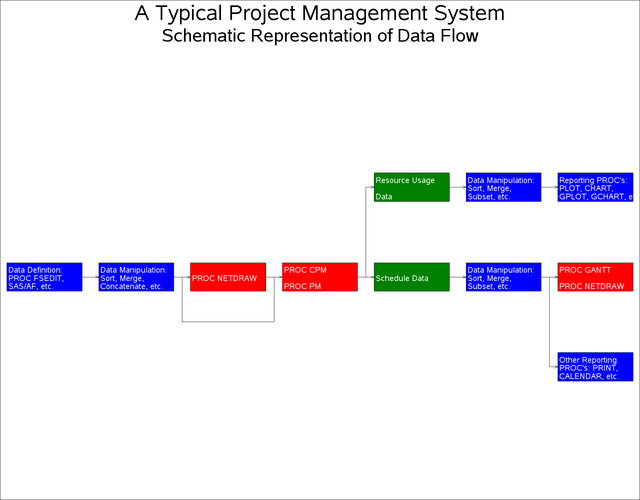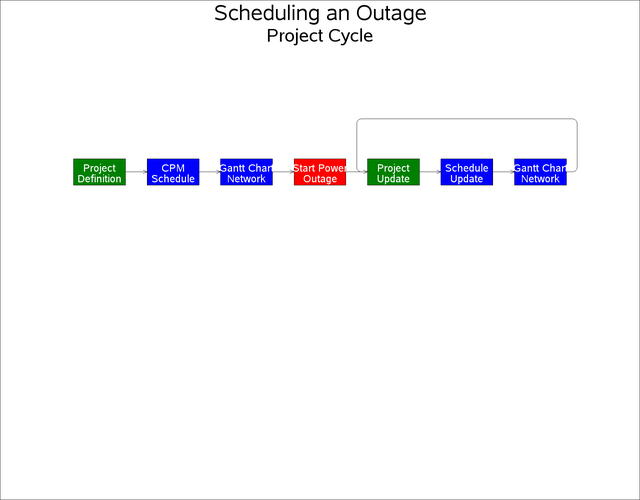Example 9.12 Schematic Diagrams

You can use PROC NETDRAW to determine node placement and arc routing for any network depicting a set of nodes connected by arcs. If you want the procedure to determine the node placement, the network must be acyclic. This example illustrates the use of PROC NETDRAW to draw two networks that represent different schematic flows. The first network does not contain any cycles, while the second one has one cycle; to draw the second network, you need to use the BREAKCYCLE option.

First, a schematic representation of the data flow going in and out of the three procedures (CPM, GANTT, and NETDRAW) is drawn using PROC NETDRAW. (See Chapter 3: Introduction to Project Management, for a detailed discussion of such a data flow.) The PATTERN= option is used to specify the variable in the data set that identifies the color that is to be used for each node. Nodes representing SAS/OR procedures are colored red, the ones representing output data sets are colored green, and all other nodes (representing the use of other parts of the SAS System) are colored blue. Three ID variables are used to specify the text that is to be placed within each node. The flow diagram is shown in Output 9.12.1.

```
data dataflow;
format id1 \$18. id2 \$14. id3 \$19. ;
input a \$ b \$ id1 & id2 & id3 & style;
datalines;
A B Data Definition:    PROC FSEDIT,    SAS/AF, etc.         2
B C Data Manipulation:  Sort, Merge,    Concatenate, etc.    2
B D Data Manipulation:  Sort, Merge,    Concatenate, etc.    2
D C .                   PROC NETDRAW    .                    1
C E PROC CPM            .               PROC PM              1
C F PROC CPM            .               PROC PM              1
E H Resource Usage      .               Data                 3
F G .                   Schedule Data   .                    3
G I Data Manipulation:  Sort, Merge,    Subset, etc.         2
G J Data Manipulation:  Sort, Merge,    Subset, etc.         2
H K Data Manipulation:  Sort, Merge,    Subset, etc.         2
I . Other Reporting     PROC's: PRINT,  CALENDAR, etc.       2
J . PROC GANTT          .               PROC NETDRAW         1
K . Reporting PROC's:   PLOT, CHART,    GPLOT, GCHART, etc.  2
;
```
```pattern1 v=s c=red;
pattern2 v=s c=blue;
pattern3 v=s c=green;

goptions hpos=110 vpos=70;
title h=3 'A Typical Project Management System';
title2 h=2.5  'Schematic Representation of Data Flow';
proc netdraw data=dataflow graphics;
actnet / act=a succ=b id = (id1-id3)
nodefaultid
nolabel
pattern=style
carcs=black coutline=black ctext=white
hmargin = 2
ybetween = 15
rectilinear
noarrowfill
pcompress htext=2;
run;
```

Output 9.12.1: Schematic Representation of Data FlowNext, a typical sequence of procedures followed at the scheduling of a nuclear power plant outage is shown using the NETDRAW procedure. Such a schematic diagram is illustrated in Chapter 3: Introduction to Project Management. In Figure 3.6, there is a cycle that is not normally allowed in a Network data set that is input to PROC NETDRAW. However, you can draw such networks by specifying the BREAKCYCLE option. (Note that you can also draw cyclic networks by specifying explicitly the node coordinates or an ALIGN= variable that fixes the x coordinates for each node.)

In this example, the data set `OUTAGE` contains the network representation. The variable `style` is used to color nodes appropriately. The resulting diagram is shown in Output 9.12.2.

```
data outage;
input a \$ b \$ id1 \$20.  id2 \$20. style;
datalines;
A   B   Project            Definition             1
B   C   CPM                Schedule               2
C   D   Gantt Chart        Network                3
D   E   Start Power        Outage                 4
E   F   Project            Update                 1
F   G   Schedule           Update                 2
G   E   Gantt Chart        Network                3
;
```
```goptions hpos=110 vpos=70;
title h=3 'Scheduling an Outage';
title2 h=2.5  'Project Cycle';

pattern1 v=s c=green;
pattern2 v=s c=blue;
pattern3 v=s c=blue;
pattern4 v=s c=red;

proc netdraw data=outage graphics;
actnet / act=a succ=b id = (id1 id2)
breakcycle
nodefaultid centerid
vmargin = 5 hmargin = 0
nolabel novcenter
pattern=style
carcs=black coutline=black ctext=white
ybetween = 15 xbetween=3
noarrowfill
pcompress htext=2;
run;
```

Output 9.12.2: Scheduling a Power Outage